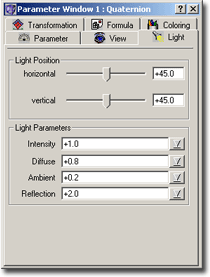### Quaternion – Light Parameters• Horizontal angle and Vertical angle

These two angles define the direction, from which the light falls onto the object.

• Intensity

This determines the intensity of the light source, so that you slowly can switch on a light source (perhaps you calculate an animation). This value must be between 0 and 1.

• Diffuse

Diffuse determines the proportion between the light from the lightsource and the reflected light. A value of 0.8 means, that 80% of the intensity of each pixel (area) is defined by the angle, in which the light from the lightsource falls on it, and 20% of the intensity by the angle, which is enclosed by the vector of the reflected light and the vector from the observer. That’s a rather crude description, I think.

To be more general, it defines, whether the 3D-picture shines due to reflected light or due to light from the lightsource.

• Ambient

‘Ambient’ is a number between 0 and 1 and determines, how much light falls on each area, independent of whether light from the lightsource actually falls on it. So it defines the minimal light intensity which falls on each pixel. A value of 1 doesn’t make much sense, because then every area would be drawn with an intensity of 1 (brightest light).

• Reflection

This parameter now determines, how strong the areas reflect the light, which falls on them. 1 means strong, 2 means less strong, 0.5 means very strong, etc.